# RS Aggarwal Class 9 Solutions Chapter 8 - Linear Equations In Two Variables

## RS Aggarwal Class 9 Chapter 8 - Linear Equations In Two Variables Solutions Free PDF

The linear equation in two variables contains two different variables in the equation which can be solved for and the generalized form can be written as ax+by=c, where x, y are variables and a,b,c are constants. The two variables must be in two separate equations, to obtain the solution of another variable.

An example of a linear equation in two variable are: 3x+5y= 6

Methods to solve linear equations in two variables are:

1. Graphing Method
2. Substitution Method
3. Elimination Method

## Download PDF of RS Aggarwal Class 9 Solutions Chapter 8 – Linear Equations In Two Variables

RS Aggarwal is one of the best reference material for Class 9 students if they want to master in mathematics. We have provided RS Aggarwal Class 9 solutions for students so that they can use it as a ready reference and prepare for their exams. The solutions are solved in a proper step by step manner for all the questions mentioned in the exercises. Solving RS Aggarwal Maths solution for Class 9 will increase the confidence level of the students.
Q.1: Draw the graph of each of the following equations:

(i) x = 5

(ii) y = -2

(iii) x + 6 = 0

(iv) x + 7 = 0

(v) y = 0

(vi) x = 0

Sol:

(i) The given equation is x = 5

For the given equation, many solutions are possible. Out of them, we take, (5, 1) and (5, -1)

Thus, forming the table:

 x 5 5 y 1 -1

On plotting the points, on the graph sheet, the following graph is obtained, in which the line AB is the required graph: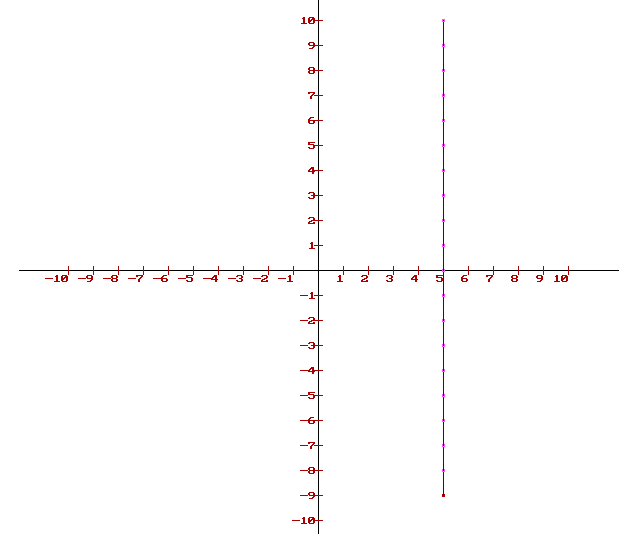(ii) The given equation is y = -2

For the given equation, many solutions are possible. Of them, we take, (1, -2) and (3, -2)

Thus, forming the table:

 x 1 3 y -2 -2

On plotting the points, on the graph sheet, the following graph is obtained, in which the line CD is the required graph: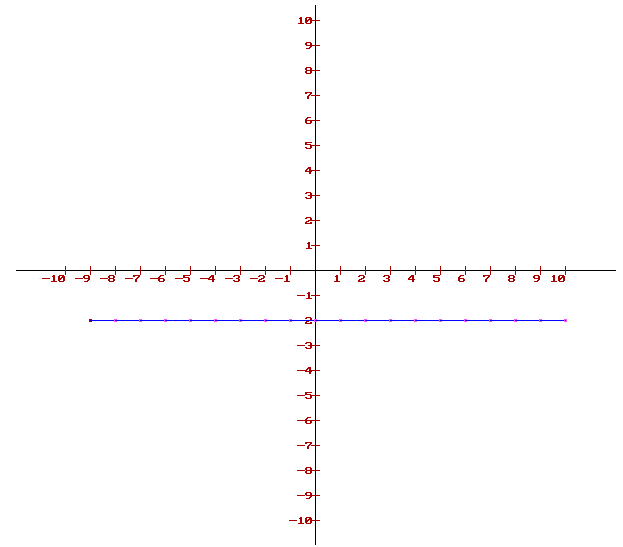(iii) The given equation is x + 6 = 0

On solving, x = -6

For the given equation, many solutions are possible. Of them, we take, (-6, 1) and (-6, -5)

Thus, forming the table:

 x -6 -6 y 1 -5

On plotting the points, on the graph sheet, the following graph is obtained, in which the line EF is the required graph: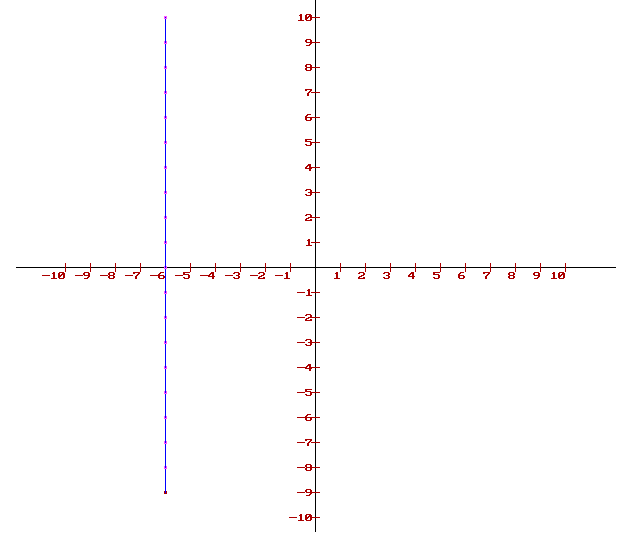(iv) The given equation is x + 7 = 0

On solving, x = -7

For the given equation, many solutions are possible. Of them, we take, (-7, 6) and (-7, -1)

Thus, forming the table, we get:

 x -7 -7 y 6 -1

On plotting the points, on the graph sheet, the following graph is obtained, in which the line GH is the required graph: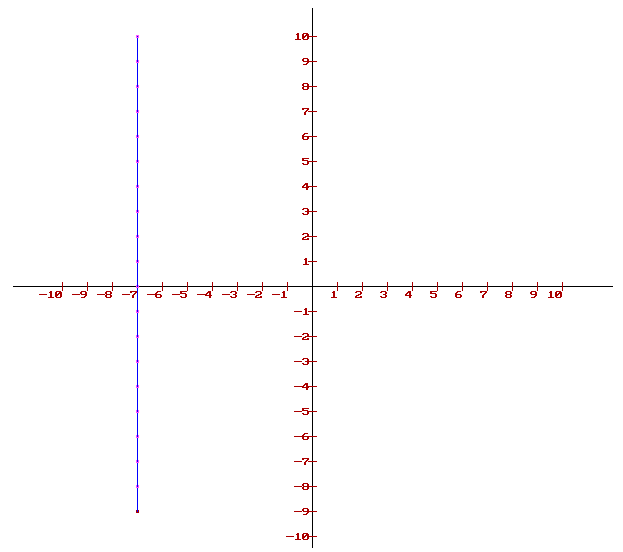(v) The given equation is x = 0, which on plotting using the above method represents the x-axis itself.

(vi) The given equation is y = 0, which on plotting using the above method represents the y-axis itself.

Q. 2: Draw the graph of the equation y = 3x. From your graph, find the value of y when x = -2.

Sol:

The given equation is y = 3x.

Assuming the values for any one variable, we can get the value for the other.

Thus, assuming the values of x as 0, 1 and 2 we get,

x = 0 $\Rightarrow$ y = 3(0) = 0

x = 1 $\Rightarrow$ y = 3(1) = 3

x = 2 $\Rightarrow$ y = 3(2) = 6

Forming the table:

 x 0 1 2 y 0 3 6

Plotting the points on the graph sheet, we obtain the required graph:

(NOTE: To draw a line, only two points is required. Here, three points are taken for better understanding)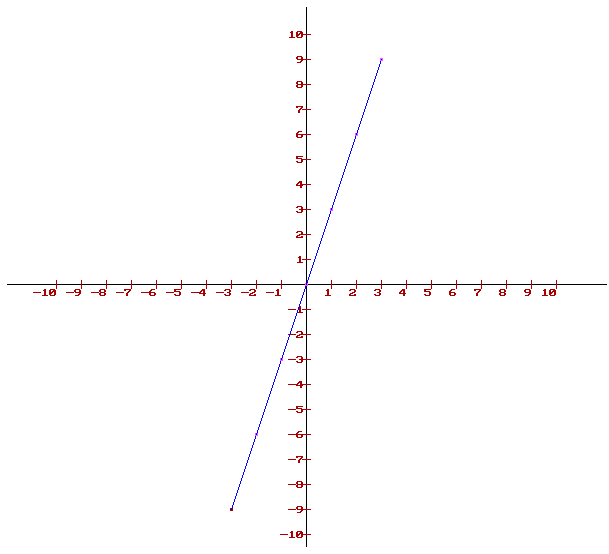Take a point A on the left of y-axis such that the distance of point A from the y-axis is 2 units.

Draw AB parallel to y-axis cutting the line y = 3x at B. Draw BC parallel to x-axis meeting y-axis at C.

Hence, y = OC = -6

Q.3: Draw the graph of the equation x + 2y – 3 = 0. Find your graph, find the value of y when x = 5.

Sol:

The given equation is x + 2y – 3 = 0.

Assuming the values for any one variable, we can get the value for the other.

Thus, assuming the values of y as -2 and 2 we get:

y = -2$\Rightarrow$ x = 3 – 2(-2) = 7

y = 2 $\Rightarrow$ x = 3 – 2(2) = -1

Forming the table:

 x 7 -1 y -2 2

Plotting the points on the graph sheet, we obtain the required graph: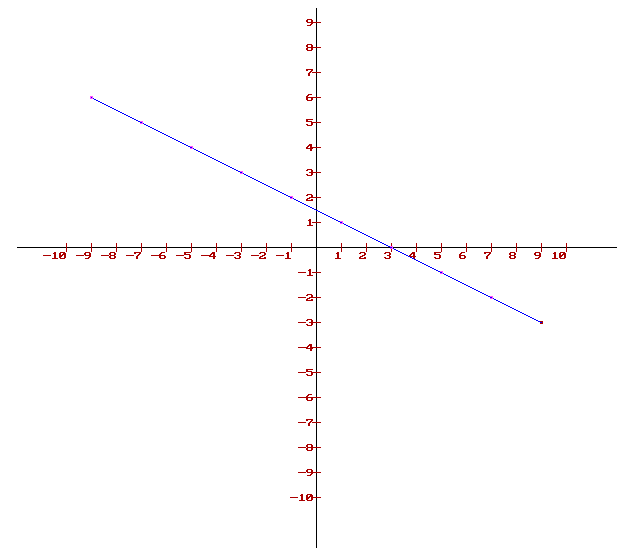Take a point P on x-axis such that OP = 5

Draw PQ parallel to y-axis cutting the line x = 3 – 2y at Q. Through Q, draw PN parallel to the x-axis at N. Hence, y = ON = -1

Q. 4: Draw the graph of each of the following equations:

(i) y = x

(ii) y = -x

(iii) y + 3x = 0

(iv) 2x + 3y = 0

(v) 3x – 2y = 0

(vi) 2x + y = 0

Sol:

(i) The given equation is y = x

Thus, when x = 1, y = 1 and when x = -1, y = -1

Thus, the table looks as:

 x 1 -1 y 1 -1

Plotting the points, the graph is obtained as follows: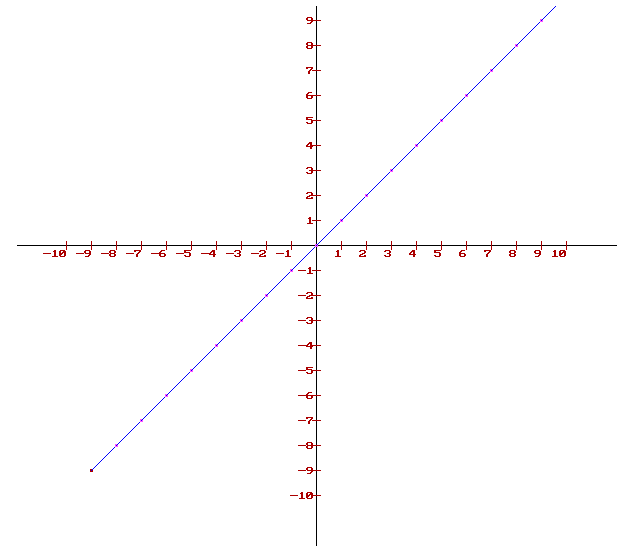(ii) The given equation is y = -x.

Thus, when x = 1, y = -1 and when x = -1, y = 1

Thus, the table looks as:

 x 1 -1 y -1 1

Plotting the points, the graph is obtained as follows: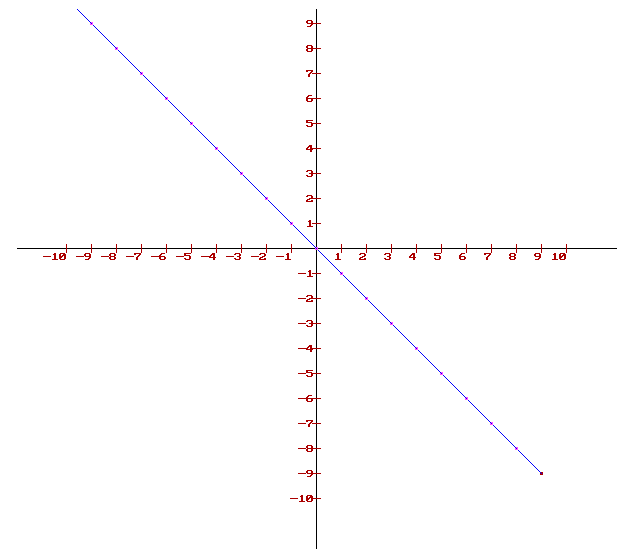(iii) The given equation is y + 3x = 0.

Solving, y = -3x

Thus, when x = 1, y = -3 and when x = -1, y = 3

Thus, the table looks as:

 x 1 -1 y -3 3

Plotting the points, the graph is obtained as follows: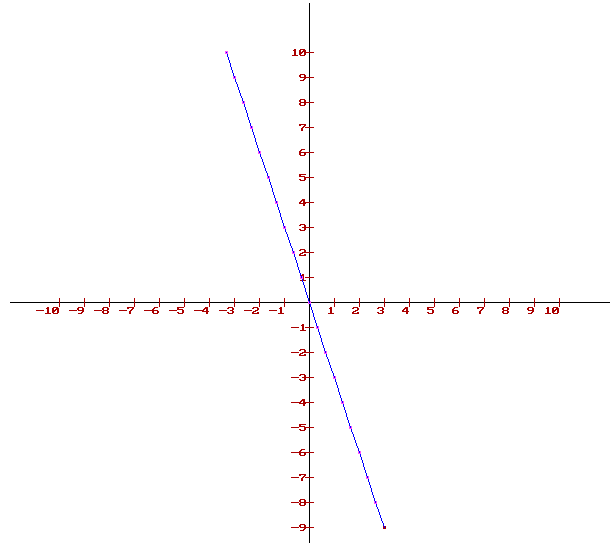(iv) The given equation is 2x + 3y = 0.

On solving, y=23×x$y\, = \, \frac{-2}{3} \, \times \, x$

Thus, when x = 3, y = -2 and when x = -3, y = 2

Thus, the table looks as:

 x 3 -3 y -2 2

Plotting the points, the graph is obtained as follows: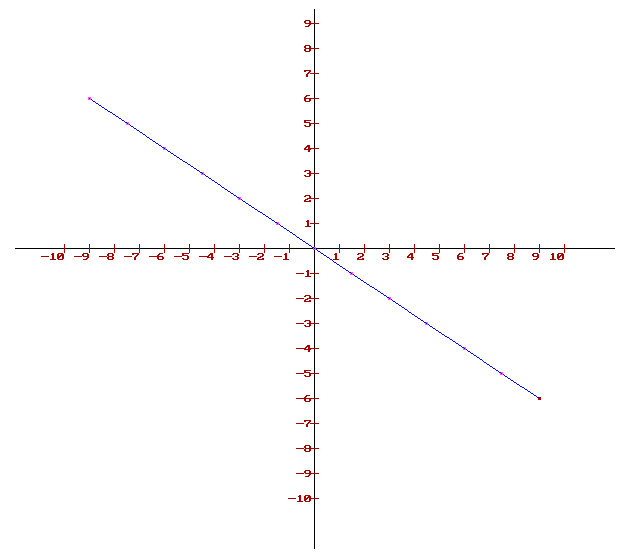(v) The given equation is 3x – 2y = 0.

On solving, y=32×x$y\, = \, \frac{3}{2} \, \times \, x$

Thus, when x = 2, y = 3 and when x = -2, y = -3

Thus, the table looks as:

 x 2 -2 y 3 -3

Plotting the points, the graph is obtained as follows: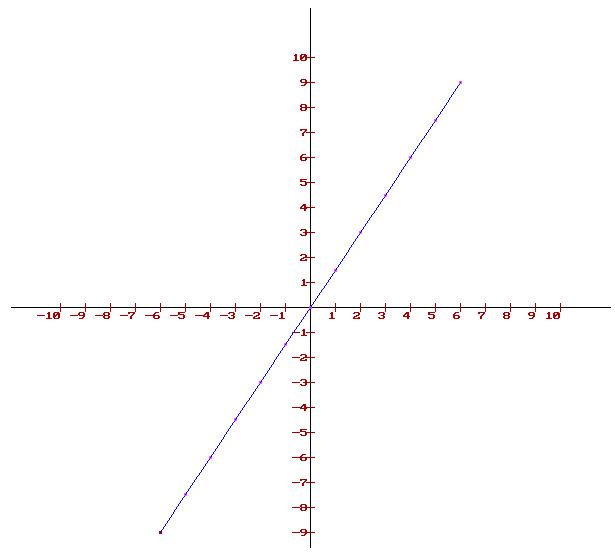(vi) The given equation is 2x + y = 0.

On solving,  y = -2x.

Thus, when x = 1, y = -2 and when x = -1, y = 2

Thus, the table looks as:

 x 1 -1 y -2 2

Plotting the points, the graph is obtained as follows: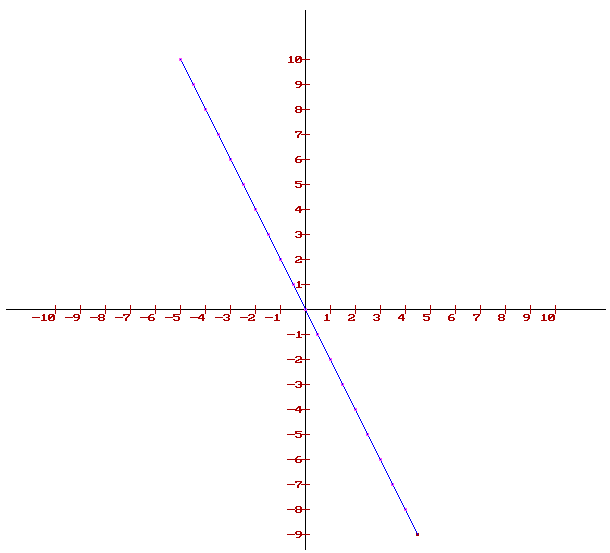Q.5: Draw the graph of the equation 2x – 3y = 5. From the graph, find (i) the value of y when x = 4, and (ii) the value of x when y = 3

Sol:

The given equation is 2x – 3y = 5.

On solving, y=2x53$y\, = \, \frac{2x – 5}{3}$

Thus, when x = 4, y = 1 and when x = -2, y = -3

Thus, the table looks as:

 x 4 -2 y 1 -3

Plotting the points, the graph is obtained as follows: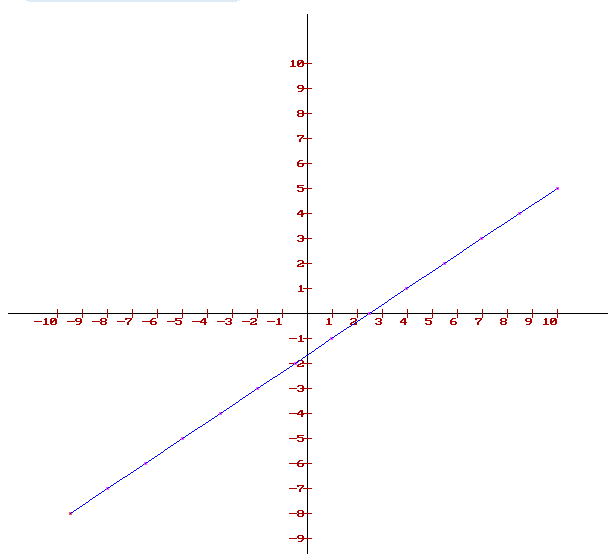Q.6: Draw the graph of the equation 2x + y = 6. Find the coordinates of the point, where the graph cuts the x-axis.

Sol:

The given equation is 2x + y = 6.

On solving, y = 6 – 2x

Thus, when x = 1, y = 4 and when x = -1, y = 8

Thus, the table looks as:

 x 1 -1 y 4 8

Plotting the points, the graph is obtained as follows: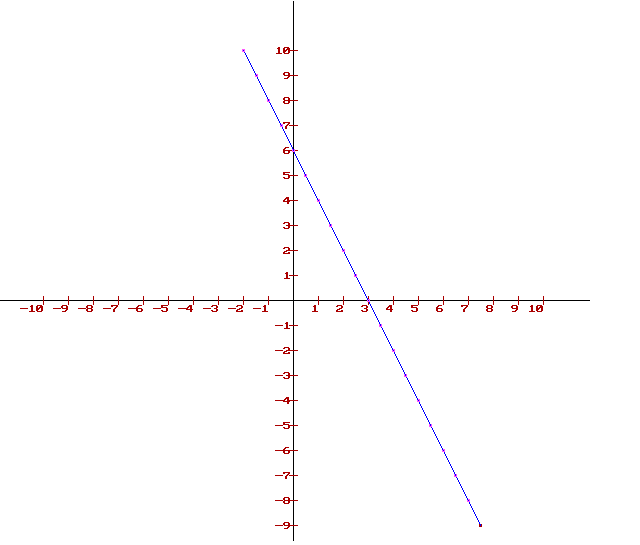We find that the line cuts the x-axis at a point P which is at a distance of 3 units to the right of the y-axis.

Therefore, the coordinates of P are (3, 0).

Q.7: Draw the graph of the equation 3x + 2y = 6. Find the coordinates of the point, where the graph cuts the y-axis.

Sol:

The given equation is 3x + 2y = 6.

On solving, y=63x2$y\, = \, \frac{6-3x}{2}$

Thus, when x = 0, y = 0 and when x = -2, y = 6

Thus, the table looks as,

 x 0 -2 y 0 6

Plotting the points, the graph is obtained as follows:

We find that the given line cuts the y-axis at a point Z which is 3 units above the x-axis.

Therefore, the coordinates of Z are (0,3).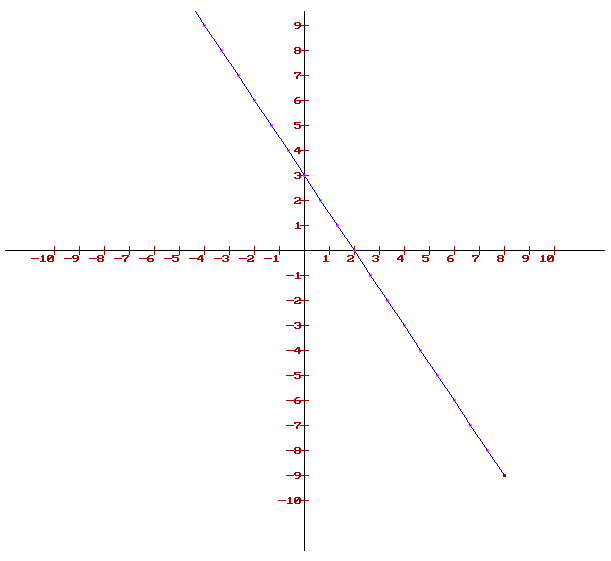CCE Questions:

Question 1:

(b) the y-axis.

X = 0 is the equation of the y-axis.

Therefore, the graph of x = 0 is YOY’

Question 2:

(a) the x-axis.

Y = 0 is the equation of x-axis.

Therefore, the graph of y = 0 is X’OX.

Question 3:

(b) parallel to the y-axis and passing through (-3, 0)

Given equation: x + 3 = 0.

Or, x = -3.

The equation of the line is parallel to the y-axis and passes through (-3, 0).

Question 4:

(b) parallel to the x-axis and passing through (0, 4)

Given equation: y – 4 = 0.

Or, y = -4.

The equation of the line parallel to the x-axis and passes through (0, 4).

Question 5:

(c) the line y = x

Given, a point of the form (a, a), where a$\neq$ 0.

When a = 1, the point is (1, 1)

When a = 2, the point is (2, 2)…. And so on.

When these points i.e., (1, 1), (2, 2)…. And so on are plotted, and the line obtained is extended on both the sides, the equation of the line y = x is obtained.

Question 6:

(d) the line x + y = 0.

Given, a point of the form (a, -a), where a$\neq$ 0.

When a = 1, the point is (1, -1)

When a = 2, the point is (2, -2)…. And so on.

When these points i.e., (1, 1), (2, 2)…. And so on are plotted, and the line obtained is extended on both the sides, the equation of line x + y = 0 is obtained.

Question 7:

(c) infinitely many solutions.

Given linear equation: 3x – 5y = 15

Or, x=5y+153$x\, = \, \frac{5y+15}{3}$

When y = 0, x = 5

When y = 3, x = 10

When y = -3, x = 0

Thus, we have the following table:

 x 5 10 0 y 0 3 -3

Plotting the points (5, 0), (10, 3) and (0, -3) and joining them and extending both the ends, we get infinite points that satisfy the given equation.

Hence, the linear equation has infinitely many solutions.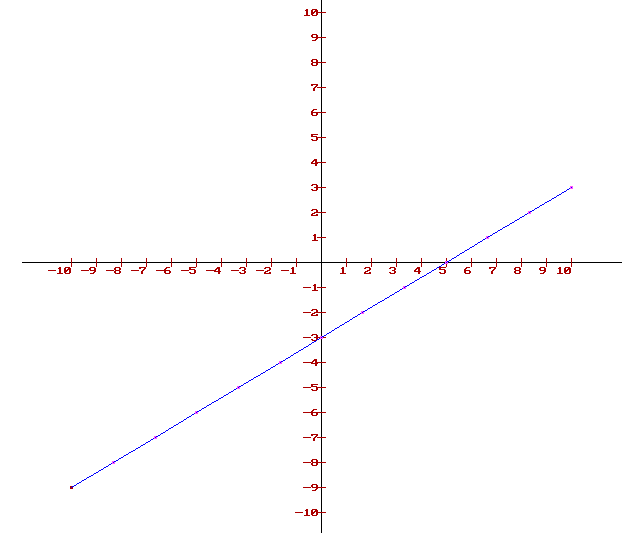Question 8:

(c) (0, 3)

Given linear equation: 3x + 2y = 6

Or, y=63x2$y\, = \, \frac{6-3x}{2}$

Putting, x = 0, we get y = 3

Thus, the graph cuts the y-axis at (0, 3)

Question 9:

(d) (3, 0)

Given equation: 4x + 3y = 12

Or, x=123y4$x\, = \, \frac{12-3y}{4}$

When, y =0, x = 3

Thus, the graph cuts the x-axis at (3, 0)

### RS Aggarwal Class 9 Solutions Chapter 8 – Linear Equations In Two Variables

Practicing questions from RS Aggarwal Maths Solution for Chapter 8 – Linear Equations In Two Variables will strengthen your knowledge of the concepts and also increase your speed. Practicing these solutions will make you feel confident while attempting the final question paper. Maths is a subject that needs lots of practice and these RS Aggarwal solutions will help you to do so as it provides different types of questions from each topic.